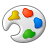# Rotate a point to the centroid of a polygon?

504
1
09-08-2017 02:58 PMNew Contributor

looking for a little help!

I'm trying to rotate a attribute point to the centroid of a parcel polygon. Ive done this manually by using rotation of point by angle. Since I have over 750 Points that change direction of flow with the change of parcel being served from time to time Id like to do a relationship between the attribute point APN and the polygon APN using the centroid of the polygon as the direction (angle of rotation) of the attribute to point in.

I'm currently working in Arc Desktop but have Arc Pro (still migrating

Tags (1)byMVP Honored Contributor

Maybe a picture would help, but here's what I'm guessing you can do:

1.) calculate centroid coordinates in polygon table

2.) calculate point coordinates in point table

3.) join polygon attributes to point attributes based on APN

4.) use good old math to calculate the angle between the point coordinates and joined centroid coordinates